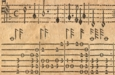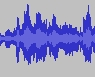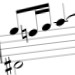# ECOLM - An Electronic Corpus of Lute Music

## Full TabCode - fingerings

Fingerings are encoded in the form `(Fhs:<pos>)`, where h is either ‘l’ or ‘r’, representing the hand to which the fingering applies, if known (this can be omitted, and normally would be, either when it is obvious from the context or it is not known), and s is a symbol from the list below.

`1`, `2`, `3`, `4` = finger-number; almost always left hand, but one or two sources where it’s right hand;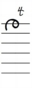Encoding: `d1(Fl4:3)`

Finger dots:Encoding: `d1(Fl.:3)`

More common: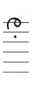Encoding: `d1(F.:7)` ("r" assumed by default)

Shorthand: `d1.`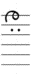Encoding: `d1(Fr..:7)`

Shorthand: `d1:`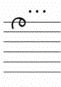Encoding: `d1(Fl...:3)`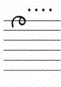Encoding: `d1(Fl....:3)` (Always left hand, as r.h. 4th finger never used.)

These indicate finger-numbers 1 to 4; usually right hand (always so when in position 7), but a number of sources use them for left-hand fingerings (in position 3); NB r.h. finger 4 is *never* used, so ‘....’ always means a left-hand fingering.

`-` = short horizontal stroke in pos 6, 7 or 8 (r.h. thumb; rare)

[Example here]

`!` = short vertical stroke in pos 7 (r.h. thumb, very common)Encoding: `d3(Fr!:7)`

Shorthand: `d3!`

`"` (i.e. a double-quote) = a pair of short vertical strokes in pos 7 (r.h. finger 2, rare)

[Example here]

Top